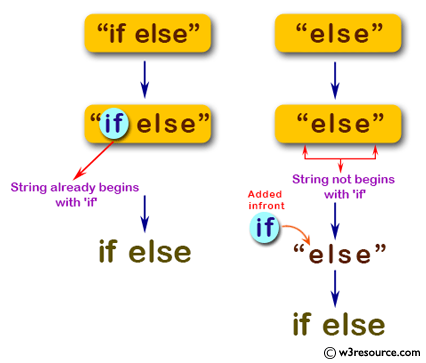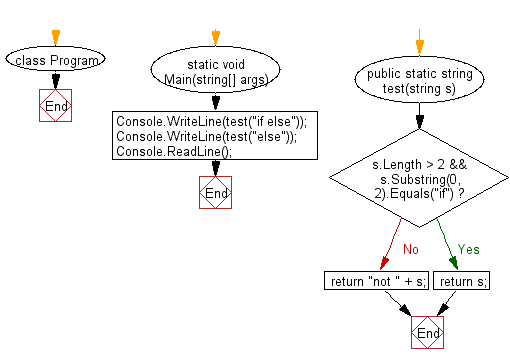﻿ C# - New string where if is added to the front of a string# C# Sharp Basic Algorithm Exercises: Create a new string where 'if' is added to the front of a given string

## C# Sharp Basic Algorithm: Exercise-5 with Solution

Write a C# Sharp program to create a new string where 'if' is added to the front of a given string. If the string already begins with 'if', return the string unchanged.

Pictorial Presentation:Sample Solution:

C# Sharp Code:

``````using System;
namespace exercises
{
class Program
{
static void Main(string[] args)
{
Console.WriteLine(test("if else"));
Console.WriteLine(test("else"));
}

public static string test(string s)
{
if (s.Length > 2 && s.Substring(0, 2).Equals("if"))
{
return s;
}
return "if " + s;
}
}
}

```
```

Sample Output:

```if else
if else```

Flowchart:C# Sharp Code Editor:

Improve this sample solution and post your code through Disqus

What is the difficulty level of this exercise?

Test your Programming skills with w3resource's quiz.

﻿